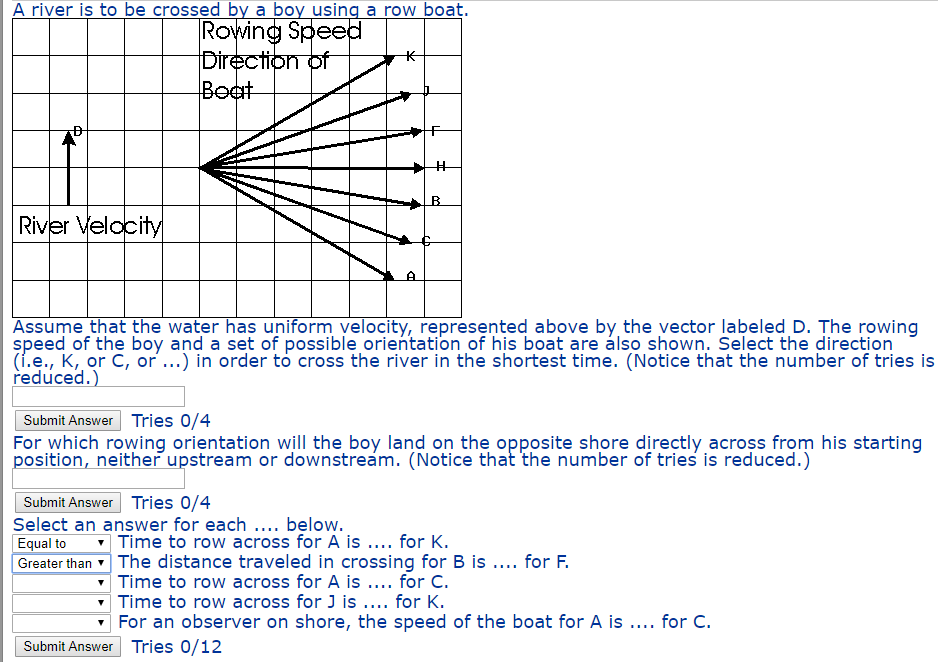# Rowing Speed tion of River Velacity Assume that the water has uniform velocity, represented above by the vector labeled...Rowing Speed tion of River Velacity Assume that the water has uniform velocity, represented above by the vector labeled D. The rowing speed of the boy and a set of possible orientation of his boat are álso shown. Select the direction (ї.е., K,or C, or ) in order to cross the river in the shortest time. (Notice that the number of tries is reduced.) Submit Answer Tries 0/4 For which rowing orientation will the boy land on the opposite shore directly across from his starting position, neither upstream or downstreám. (Notice that the number of tries is reduced.) Submit Answer Tries 0/4 Select an answer for each.... below. Equal toTime to row across for A is for K. Greater than The distance traveled in crossing for B is.... for F Time to row across for A is for C. Time to row across forJ is... for K. For an observer on shore, the speed of the boat for A is.. for C Submit Answer Tries 0/12

Concept used - Aiming with the current sweeps her much farther downstream than aiming against the current

----------------------------------------------------------------------------

direction to cross the river in shortest time is H

--------------------------------------------------------------------------------

Boy will land on the opposite shore for direction C

-------------------------------------------------------------------------------------------

Time to row across for A is equal to for K

The distance traveled in crossing for B is less than for F

time to row across for A is more than for C

time to row across for J is less than K

For an observer on shore, the speed of boat for A is more than for C (not sure about this one)

##### Add Answer of: Rowing Speed tion of River Velacity Assume that the water has uniform velocity, represented above by the vector labeled...
More Homework Help Questions Additional questions in this topic.